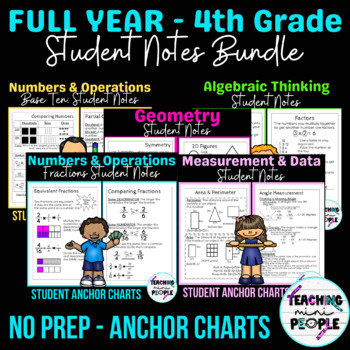# 4th Grade Math Notes | Full Year Bundle

Rated 4.84 out of 5, based on 29 reviews
29 Ratings;
4th - 5th, Homeschool
Subjects
Resource Type
Standards
Formats Included
• Zip
Pages
60+
\$13.50
List Price:
\$17.00
You Save:
\$3.50
Bundle
\$13.50
List Price:
\$17.00
You Save:
\$3.50
Bundle
Report this resource to TPT

#### What educators are saying

These are great for interactive notebooks, and allow my students to reference HOW to complete problems. I love how the steps are broken down in multiple ways! Thank you!

#### Bonus

All in One File

### Description

This 4th Grade Math Anchor Chart Bundle accompanies the 4th grade math standards. Students can glue these 4th grade math anchor charts into their interactive notebooks to have and refer to all year. These can also be great for small group review, ELL learners, resource students or messy writers to help keep them organized during math.

TOPICS INCLUDED:

*Numbers & Operations in Base Ten

• Place Value Word Splash
• Place Value Chart
• Place VS Value
• 10x the Value
• Place Value Forms
• Expanded Form
• Word Form
• Compare Numbers
• Rounding Rap/Saying
• Rounding to TENS on a Number Line
• Rounding to HUNDREDS on a Number Line
• Rounding on a Number Line Visual
• Estimating Real World
• Add & Subtract Large Numbers
• Multiplication Strategies
• Partial Products
• Partial Quotient
• Long Division
• Word Problem Key Words

*Operations & Algebraic Thinking

• Multiplicative Comparison
• Do I multiply or divide?
• Factors
• Multiples
• GCF & LCM
• Patterns – In/Out Tables

*Number & Operations – Fractions

• Fractions
• Simplifying Fractions
• Equivalent Fractions
• Common Denominators
• Comparing Fractions
• Add & Subtract Fractions (Like denominators)
• Add & Subtract Fractions (Unlike denominators)
• Multiply Fractions by Whole Numbers
• Fractions w/ Denominators 10 & 100
• Decimals
• Fractions Decimals
• Comparing Decimals

*Geometry

• Lines Vocabulary
• Angles
• Triangles
• 2D Figures
• Symmetry

*Measurement & Data

• What is measurement?
• Relative Sizes: Length
• Relative Sizes: Capacity/Volume
• Relative Sizes: Weight/Mass
• Customary Measurements
• Mr. Gallon Man
• Metric Measurements
• How to Read Line Plots
• Creating a Line Plot
• Line Plot Key Words
• Sunflower Line Plot
• Perimeter
• Area
• Irregular Area
• Comparing Area & Perimeter
• Angle Measurements
• Degrees in a circle

**The preview only shows some of the anchor charts per set.

What’s Included:

➜ 70+ pages

➜ Interactive math anchor charts for the above mentioned topics

NEED MORE ACTIVITIES LIKE THIS?:

4th Grade Mid Year Math Review *EDITABLE*

6th Grade Math Anchor Charts Growing BUNDLE - Reference Sheets - Notebook

End of 4th Grade Math Review - Colored Jenga

Math Assessments NO PREP - FULL YEAR

Ratios & Proportions Math Anchor Charts - Student Reference Sheets - 6th

FIND ME HERE:

Instagram

Blog

Email

❤️ Make sure to leave a review to receive credits for future purchases - THAT'S FREE CREDITS THAT YOU CAN USE FOR FUTURE TPT PURCHASES!!

❤️ If you have any questions or experience a problem, please send me an email or ask on the Q&A page prior to leaving a review. I will respond as soon as possible to all questions!

Total Pages
60+
Included
Teaching Duration
1 Year
Report this resource to TPT
Reported resources will be reviewed by our team. Report this resource to let us know if this resource violates TPT’s content guidelines.

### Standards

to see state-specific standards (only available in the US).
Know relative sizes of measurement units within one system of units including km, m, cm; kg, g; lb, oz.; l, ml; hr, min, sec. Within a single system of measurement, express measurements in a larger unit in terms of a smaller unit. Record measurement equivalents in a two-column table. For example, know that 1 ft is 12 times as long as 1 in. Express the length of a 4 ft snake as 48 in. Generate a conversion table for feet and inches listing the number pairs (1, 12), (2, 24), (3, 36),...
Use the four operations to solve word problems involving distances, intervals of time, liquid volumes, masses of objects, and money, including problems involving simple fractions or decimals, and problems that require expressing measurements given in a larger unit in terms of a smaller unit. Represent measurement quantities using diagrams such as number line diagrams that feature a measurement scale.
Apply the area and perimeter formulas for rectangles in real world and mathematical problems. For example, find the width of a rectangular room given the area of the flooring and the length, by viewing the area formula as a multiplication equation with an unknown factor.
Make a line plot to display a data set of measurements in fractions of a unit (1/2, 1/4, 1/8). Solve problems involving addition and subtraction of fractions by using information presented in line plots. For example, from a line plot find and interpret the difference in length between the longest and shortest specimens in an insect collection.
Recognize angles as geometric shapes that are formed wherever two rays share a common endpoint, and understand concepts of angle measurement: